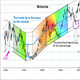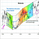English# Gann's angle ratios (Can't set it perfect for Gann)

879 views
879
Here you go. The angle ratios to go with the angles squares. Everything is geometrically tied to together. That 45 degree line is actually 33.69 degrees. Just draw 90 degree diagonals and you'll see, the chart isn't a 1/1 scale it's 1/1.5 scale.This 1/1 angle is actually 53.1 degrees. Gann idea probably works but the chart scale is .66666... The scale on this chart is actually 1/1.5 so the Gann tool doesn't work out as far as I can see. If you check my other chart where I draw a 90 angle to test the scale you'll see.The Gann angles are set on 1/1 or 45 degrees from the top but that actual angle because of the scale of the chart is 56.31 degrees down from the right angle of the square top. I applied the scale to calculate the actual angle from an equation I use in my TI-85.
(Y-Y1)=(tan (T))*SC(X-X1)POWER (1) SC is the scale. T is the actual angle in degrees.I set the squares to follow the actual data, but the squares might not be perfectly square. Anyway the idea is there.I know what it is. It starts at the top on the 1/8 line. That should be 0.
EN English
EN English (UK)
EN English (IN)
DE Deutsch
FR Français
ES Español
IT Italiano
PL Polski
SV Svenska
TR Türkçe
RU Русский
PT Português
ID Bahasa Indonesia
MS Bahasa Melayu
TH ภาษาไทย
VI Tiếng Việt
JA 日本語
KO 한국어
ZH 简体中文
ZH 繁體中文
AR العربية
HE עברית